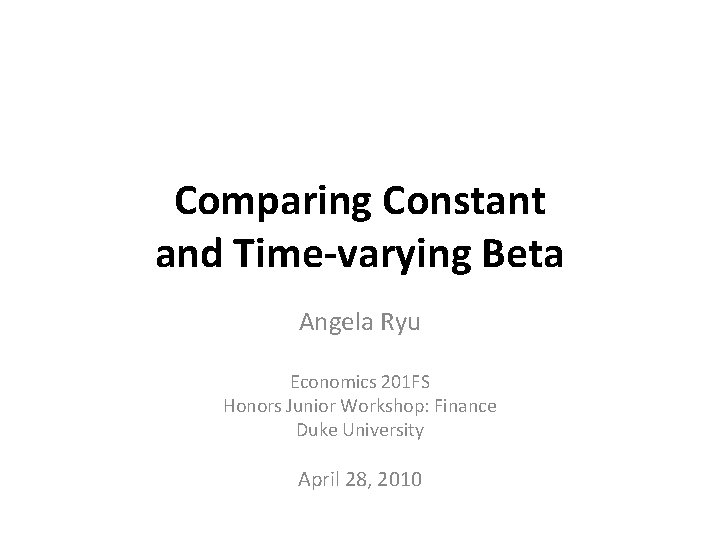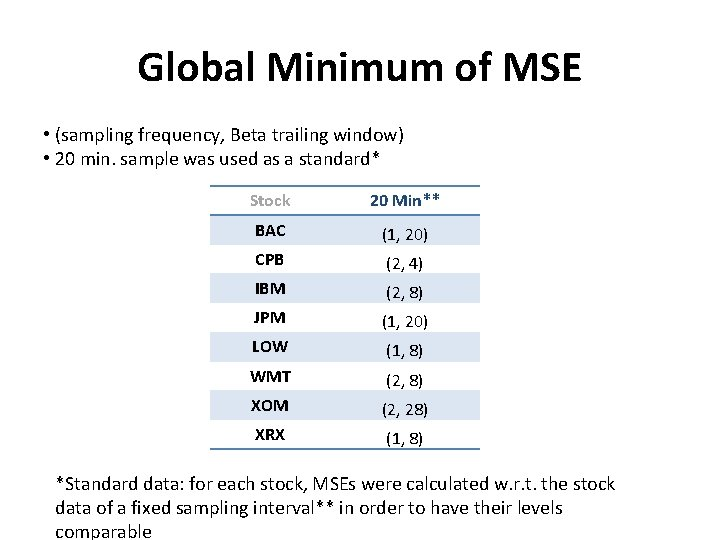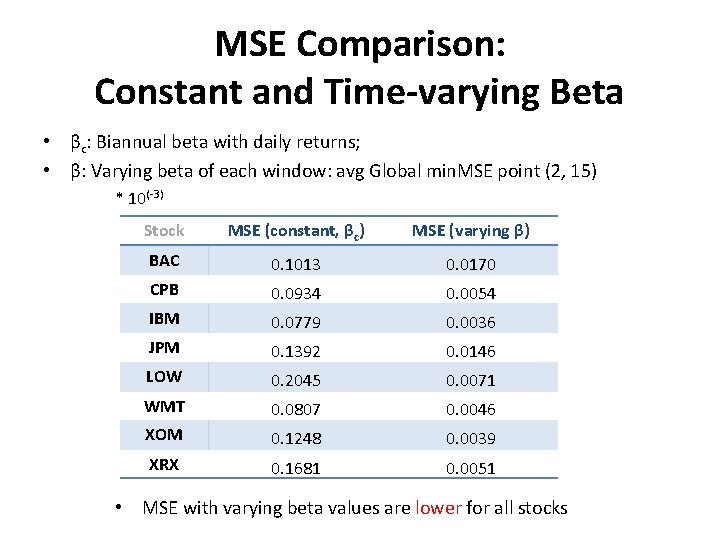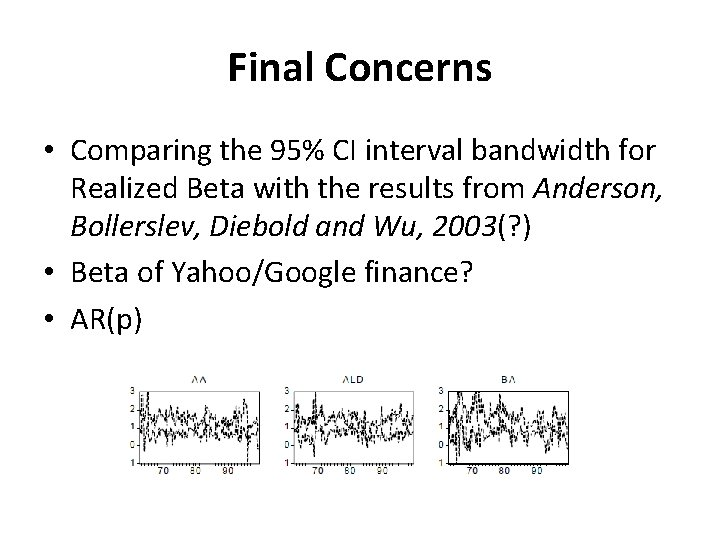# Comparing Constant and Timevarying Beta Angela Ryu Economics

• Slides: 11
Download presentationComparing Constant and Time-varying Beta Angela Ryu Economics 201 FS Honors Junior Workshop: Finance Duke University April 28, 2010Data • BAC, CPB, IBM, JPM, LOW, WMT, XOM, XRX • SPY • Data from Aug 23 2004 – Jan 7 2009 (1093 days) • Sampling interval: 1 – 20 min. • Beta trailing window: 1 – 200 days Notation: – (s, w) = (Sampling interval, Beta window) – g. Min(s, w) = (s, w) that yields global minimum MSE for given stockSummary of Previous Work • Goal: Empirically find an optimal (s, w) that yields minimum MSE. • Found g. Min(s, w) of each stocks, w varying from 1 – 50. Relatively short span for total 1093 days of data, so extension of windows is necessary. • Observed that g. Min(s, w) is at higher frequency (1, 2 min interval) with relatively short trailing window. (Given short interval s, longer w than w of g. Min yielded higher MSEs. ) • Concluded that there is an optimality in calculating Beta with recent data of high frequency despite Microstructure noise.Additional Computation • Extend the range of w to 1 - 200, plot 3 D distribution of MSE and find g. Min(s, w) • Plot Realized Beta of each stock with s, w of g. Min. (Anderson, Bollerslev, Diebold and Wu, 2003) • Compare MSE levels using 1) constant beta and 2) time-varying beta, attained from g. Min(s, w)3 D plotGlobal Minimum of MSE • (sampling frequency, Beta trailing window) • 20 min. sample was used as a standard* Stock 20 Min** BAC (1, 20) CPB (2, 4) IBM (2, 8) JPM (1, 20) LOW (1, 8) WMT (2, 8) XOM (2, 28) XRX (1, 8) *Standard data: for each stock, MSEs were calculated w. r. t. the stock data of a fixed sampling interval** in order to have their levels comparableTime-series plot of Realized Beta BAC LOW CPB WMT IBM XOM • Used 2 min. , 15 days • 1078 (=1093 – 15) observations JPM XRXMSE Comparison: Constant and Time-varying Beta • βc: Biannual beta with daily returns; • β: Varying beta of each window: avg Global min. MSE point (2, 15) * 10(-3) Stock MSE (constant, βc) MSE (varying β) BAC 0. 1013 0. 0170 CPB 0. 0934 0. 0054 IBM 0. 0779 0. 0036 JPM 0. 1392 0. 0146 LOW 0. 2045 0. 0071 WMT 0. 0807 0. 0046 XOM 0. 1248 0. 0039 XRX 0. 1681 0. 0051 • MSE with varying beta values are lower for all stocksResults • Unlike our intuition, despite microstructure noise, global minimum of MSEs of all pairs of (s, w) appeared at the highest frequency. • More days in the trailing window did not compensate the loss of data due to lower sampling frequency, even longer than half a year (w >126) • MSE levels was lower in time-varying beta case, but choosing the constant beta was made arbitrarily which remains as a problemConclusion • Using high-frequency data, we empirically found a time-varying beta of some sampling frequency and trailing window that performs better than a constant beta in terms of the level of MSE • Even in obvious presence of microstructure noise, high frequency with some threshold range of trailing window gave the least MSE, suggesting that high-frequency data gives a relatively better prediction of beta • However, using time-varying beta may hurt the prediction even more than does using a constant beta if misspecified (Ghysels, 1998) ; thus comparison with constant beta should be made more carefully.Final Concerns • Comparing the 95% CI interval bandwidth for Realized Beta with the results from Anderson, Bollerslev, Diebold and Wu, 2003(? ) • Beta of Yahoo/Google finance? • AR(p)